Home Practice
For learners and parents For teachers and schools
Textbooks
Full catalogue
Pricing SupportLog in

We think you are located in United States. Is this correct?

# 4.5 Applications of organic chemistry

## 4.5 Applications of organic chemistry (ESCKV)

### Alkanes as fossil fuels (ESCKW)

Fossil fuels are fuels formed by the natural process of the decomposition of organisms under heat and pressure. They contain a high percentage of carbon and include fuels such as coal, petrol, and natural gases. They are also a non-renewable.

Hydrocarbon cracking

Hydrocarbon cracking is the process of breaking carbon-carbon bonds in long-chain hydrocarbons to form simpler, shorter-chain hydrocarbons.

Hydrocarbon cracking is an important industrial process. Through this process long, bulky alkanes are broken up into smaller compounds. These compounds include shorter alkanes and alkenes. A few examples of cracking hydrocarbons are given in Figure 4.69.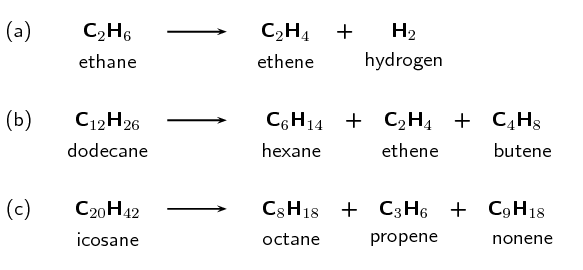Figure 4.69: The cracking of various hydrocarbons to produce alkanes and alkenes.

There are two types of hydrocarbon cracking. Thermal cracking occurs under high pressures and temperatures without a catalyst, catalytic cracking occurs at lower pressures and temperatures in the presence of a catalyst. This process is a common source of shorter (more useful) alkanes as well as unsaturated alkenes. The alkanes are then used in combustion processes.

It is possible to separate the products of hydrocarbon cracking and obtain specific products from a crude oil mix through a process called fractional distillation. This is done using a fractionating column. Crude oil evaporates when heated to $$\text{700}$$$$\text{°C}$$. The gas bubbles through a tray that is kept at a certain temperature. The alkanes and alkenes that condense at that temperature will then condense in the tray. For example if the tray is kept at $$\text{170}$$$$\text{°C}$$ the product will be paraffin oil (Figure 4.70).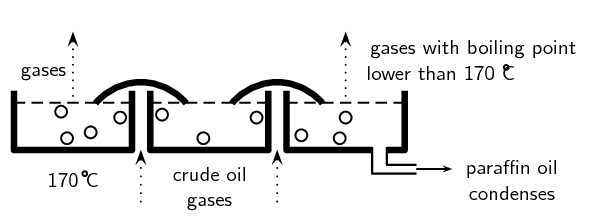Figure 4.70: One layer of a fractionating column. Image by Duncan Watson.

A fractionating column has a series of these trays (Figure 4.71), each at a constant temperature. This means that many compounds can be separated from the crude oil mix. The crude oil is heated to $$\text{700}$$$$\text{°C}$$ and the gas of the crude oil is passed through the column. Bitumen for tar roads is collected at the bottom of the fractionating column. These are all compounds with more than $$\text{70}$$ carbon atoms. The temperature decreases as you move up the column. As the gases rise, compounds with different length carbon chains condense until only the chains with $$\text{1}$$ - $$\text{4}$$ carbon atoms are collected at the top of the column. These are used for liquid petroleum gas.

For more information on the process of fractional distillation have a look at this animation:

Note that this fits with what we learned in the previous section. The more carbon atoms in the chain, the greater the intermolecular forces and therefore the higher the boiling point. That means that these molecules will condense at higher temperatures.

Combustion of alkanes

Alkanes are our most important fossil fuels. The combustion (burning) of alkanes (also known as oxidation) is highly exothermic.

Combustion

In a combustion reaction a substance reacts with an oxidising agent (e.g. oxygen), and heat and light are released.

In the complete combustion reaction of alkanes, carbon dioxide ($$\text{CO}_{2}$$) and water ($$\text{H}_{2}\text{O}$$) are released along with energy. Fossil fuels are burnt for the energy they release. The general reaction for the combustion of an alkane as a fossil fuel is given in Figure 4.72

The complete combustion of alkanes produces only $$\text{CO}_{2}$$ and $$\text{H}_{2}\text{O}$$. Not all combustion process are complete though. An incomplete combustion will also produce carbon monoxide ($$\text{CO}$$).

Remember that an exothermic reaction releases energy ($$\Delta \text{H} < \text{0}$$), while an endothermic reaction absorbs energy ($$\Delta \text{H} > \text{0}$$). The fact that energy is released in a combustion reaction implies that $$\Delta \text{H} < \text{0}$$ and the reaction is exothermic.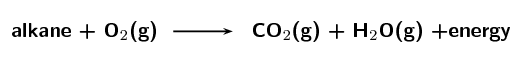Figure 4.72: The complete combustion of an alkane.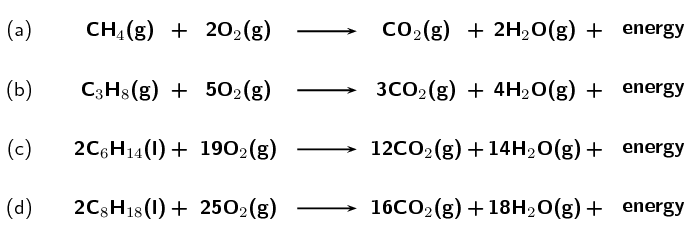Figure 4.73: The complete combustion reactions of (a) methane, (b) propane, (c) hexane and (d) octane.

## Worked example 30: Balancing equations

Balance the following equation:

$$\text{C}_{4}\text{H}_{10}(\text{g}) + \text{O}_{2}(\text{g})$$ $$\to$$ $$\text{CO}_{2}(\text{g}) + \text{H}_{2}\text{O}(\text{g})$$

### Balance the carbon atoms

There are 4 carbon atoms on the left. There is 1 carbon atom on the right. Add a 4 in front of the $$\text{CO}_{2}$$ molecule on the right:

$$\text{C}_{4}\text{H}_{10}(\text{g}) + \text{O}_{2}(\text{g})$$ $$\to$$ $$4\text{CO}_{2}(\text{g}) + \text{H}_{2}\text{O}(\text{g})$$

### Balance the hydrogen atoms

There are 10 hydrogen atoms on the left. There are 2 hydrogen atoms on the right. Add a 5 in front of the $$\text{H}_{2}\text{O}$$ molecule on the right:

$$\text{C}_{4}\text{H}_{10}(\text{g}) + \text{O}_{2}(\text{g})$$ $$\to$$ $$4\text{CO}_{2}(\text{g}) + 5\text{H}_{2}\text{O}(\text{g})$$

### Balance the oxygen atoms

There are 2 oxygen atoms on the left. There are 13 oxygen atoms on the right ($$\text{4}$$ x $$\text{2}$$ in the $$\text{CO}_{2}$$ and 5 in the $$\text{H}_{2}\text{O}$$). Divide the number of $$\text{O}$$ atoms on the right by $$\text{2}$$ to get $$\frac{13}{2}$$, this is the number of $$\text{O}_{2}$$ molecules required on the left:

$$\text{C}_{4}\text{H}_{10}(\text{g}) +$$$$\frac{13}{2}$$$$\text{O}_{2}(\text{g})$$ $$\to$$ $$4\text{CO}_{2}(\text{g}) + 5\text{H}_{2}\text{O}(\text{g})$$

This is acceptable but it is better for all numbers to be whole numbers.

### Make sure all numbers are whole numbers

There is $$\frac{13}{2}$$ in front of the $$\text{O}_{2}$$ while all other numbers are whole numbers. So multiply the entire equation by 2:

$$2\text{C}_{4}\text{H}_{10}(\text{g}) + 13\text{O}_{2}(\text{g})$$ $$\to$$ $$8\text{CO}_{2}(\text{g}) + 10\text{H}_{2}\text{O}(\text{g})$$

## Worked example 31: Balancing equations

Balance the equation for the complete combustion of heptane

### Write the unbalanced equation

The molecular formula for heptane is $$\text{C}_{7}\text{H}_{16}$$. Combustion always involves oxygen ($$\text{O}_{2}$$). The complete combustion of an alkane always produces carbon dioxide ($$\text{CO}_{2}$$) and water ($$\text{H}_{2}\text{O}$$):

$$\text{C}_{7}\text{H}_{16}(\text{ℓ}) + \text{O}_{2}(\text{g})$$ $$\to$$ $$\text{CO}_{2}(\text{g}) + \text{H}_{2}\text{O}(\text{g})$$

### Balance the carbon atoms

There are 7 carbon atoms on the left. There is 1 carbon atom on the right. Add a 7 in front of the $$\text{CO}_{2}$$ molecule on the right:

$$\text{C}_{7}\text{H}_{16}(\text{ℓ}) + \text{O}_{2}(\text{g})$$ $$\to$$ $$7\text{CO}_{2}(\text{g}) + \text{H}_{2}\text{O}(\text{g})$$

### Balance the hydrogen atoms

There are 16 hydrogen atoms on the left. There are 2 hydrogen atoms on the right. Add an 8 in front of the $$\text{H}_{2}\text{O}$$ molecule on the right:

$$\text{C}_{7}\text{H}_{16}(\text{ℓ}) + \text{O}_{2}(\text{g})$$ $$\to$$ $$7\text{CO}_{2}(\text{g}) + 8\text{H}_{2}\text{O}(\text{g})$$

### Balance the oxygen atoms

There are 2 oxygen atoms on the left. There are 22 oxygen atoms on the right ($$\text{7}$$ x $$\text{2}$$ in the $$\text{CO}_{2}$$ and 8 in the $$\text{H}_{2}\text{O}$$). Divide the number of $$\text{O}$$ atoms on the right by $$\text{2}$$ to get $$\text{11}$$, this is the number of $$\text{O}_{2}$$ molecules required on the left:

$$\text{C}_{7}\text{H}_{16}(\text{ℓ}) + 11\text{O}_{2}(\text{g})$$ $$\to$$ $$7\text{CO}_{2}(\text{g}) + 8\text{H}_{2}\text{O}(\text{g})$$

## Alkanes as fossil fuels

Textbook Exercise 4.24

Balance the following complete combustion equations:

$$\text{C}_{3}\text{H}_{8}(\text{g}) + \text{O}_{2}(\text{g})$$ $$\to$$ $$\text{CO}_{2}(\text{g}) + \text{H}_{2}\text{O}(\text{g})$$

$$\text{C}_{3}\text{H}_{8}(\text{g}) + 5\text{O}_{2}(\text{g})$$ $$\to$$ $$3\text{CO}_{2}(\text{g}) + 4\text{H}_{2}\text{O}(\text{g})$$

$$\text{C}_{7}\text{H}_{16}(\text{ℓ}) + \text{O}_{2}(\text{g})$$ $$\to$$ $$\text{CO}_{2}(\text{g}) + \text{H}_{2}\text{O}(\text{g})$$

$$\text{C}_{7}\text{H}_{16}(\text{ℓ}) + 11\text{O}_{2}(\text{g})$$ $$\to$$ $$7\text{CO}_{2}(\text{g}) + 8\text{H}_{2}\text{O}(\text{g})$$

$$\text{C}_{2}\text{H}_{6}(\text{g}) + \text{O}_{2}(\text{g})$$ $$\to$$ $$\text{CO}_{2}(\text{g}) + \text{H}_{2}\text{O}(\text{g})$$

$$2\text{C}_{2}\text{H}_{6}(\text{g}) + 7\text{O}_{2}(\text{g})$$ $$\to$$ $$4\text{CO}_{2}(\text{g}) + 6\text{H}_{2}\text{O}(\text{g})$$

Write the balanced equation for the complete combustion of:

octane

$$2\text{C}_{8}\text{H}_{18}(\text{ℓ}) + 25\text{O}_{2}(\text{g})$$ $$\to$$ $$16\text{CO}_{2}(\text{g}) + 18\text{H}_{2}\text{O}(\text{g})$$

pentane

$$\text{C}_{5}\text{H}_{12}(\text{ℓ}) + 8\text{O}_{2}(\text{g})$$ $$\to$$ $$5\text{CO}_{2}(\text{g}) + 6\text{H}_{2}\text{O}(\text{g})$$

hexane

$$2\text{C}_{6}\text{H}_{14}(\text{ℓ}) + 19\text{O}_{2}(\text{g})$$ $$\to$$ $$12\text{CO}_{2}(\text{g}) + 14\text{H}_{2}\text{O}(\text{g})$$

butane

$$2\text{C}_{4}\text{H}_{10}(\text{g}) + 13\text{O}_{2}(\text{g})$$ $$\to$$ $$8\text{CO}_{2}(\text{g}) + 10\text{H}_{2}\text{O}(\text{g})$$

Is the combustion of alkanes exothermic or endothermic? What does that mean?

It is exothermic. This means that energy is released during the combustion of an alkane.

### Esters (ESCKX)

#### Production of esters

As was discussed earlier in this chapter one way to form an ester is through the reaction of an alcohol and a carboxylic acid. This process is called an acid-catalysed condensation or esterification of a carboxylic acid.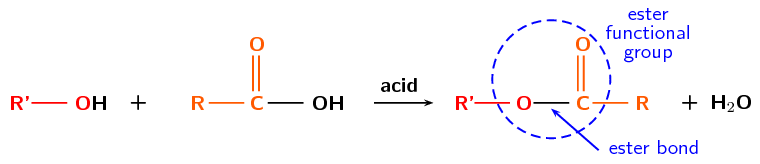Figure 4.74: The acid-catalysed condensation of a carboxylic acid to form an ester.

In the formation of an ester one oxygen atom comes from the alcohol molecule, while the carbonyl group comes from the carboxylic acid. This is known because it is possible to label (using radioactive nuclides) the atoms of the reactants and see where they end up in the products.

In the general form above an alcohol (red) and a carboxylic acid (orange) combine to form an ester and water. A specific example is given in Figure 4.75 for the formation of butyl propanoate and water.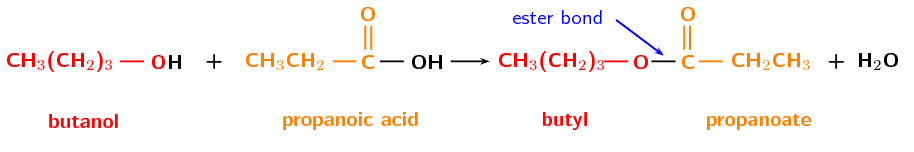Figure 4.75: The esterification of butanol and propanoic acid to form butyl propanoate, water is also formed in this reaction.

This reaction can also be written as: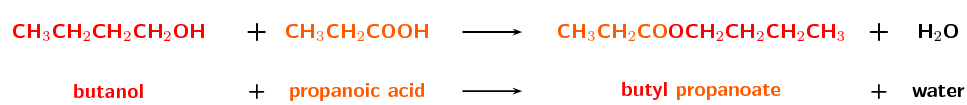A more general example is: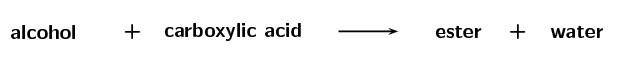It is important to be able to identify what ester a specific alcohol and carboxylic acid will form. Remember that the first part of the ester name takes its prefix from the alcohol with the suffix -yl. The second part of the ester takes its prefix from the carboxylic acid with the ester suffix -oate.

## Worked example 32: Determining ester names

What is the name of the ester that will form from hexanol and propanoic acid

### Which compound forms the first part of the ester name and which forms the second part of the ester name?

The alcohol forms the first part of the ester name and takes the suffix -yl. The carboxylic acid forms the second part of the ester name and takes the suffix -oate.

### Determine the first part of the ester name

The alcohol is hexanol, therefore there are 6 carbons and this will be hexyl.

### Determine the second part of the ester name

The carboxylic acid is propanoic acid, therefore there are 3 carbons and this will be propanoate.

### Combine the first and second parts of the ester name

The ester will be hexyl propanoate.

It is also important to be able to determine which compounds were used to form an ester.

## Worked example 33: Determining starting materials of esters

What compounds did the ester octyl heptanoate come from?

### What types of compounds are used to form esters?

Esters are formed from alcohols (which become the first part of the ester name) and carboxylic acids (which become the second part of the ester name).

### Determine the prefix for the alcohol

The first part of the ester name comes from the alcohol (-ol). Therefore the prefix is oct-.

### Determine the prefix for the carboxylic acid

The second part of the ester name comes from the carboxylic acid (-oic acid). Therefore the prefix is hept-.

### Determine the compounds use to form the ester

Octyl heptanoate was formed from octanol and heptanoic acid.

A few examples of esters are given in Table 4.17.

 Ester name Smell Uses methyl methanoate ether quick-dry finishes insecticide pharmaceuticals ethyl methanoate rum lacquers safety glass fumigating foods propyl methanoate pears solvent flavour fragrance methyl ethanoate glue glues paints nail polish remover ethyl ethanoate apple glues etc solvents decaffeination propyl ethanoate pear solvent flavour fragrance butyl ethanoate banana or apple lacquers flavour pentyl ethanoate banana or apple lacquers solvents resins methyl butanoate apple or pineapple flavour fragrance ethyl butanoate pineapple flavour fragrance plasticiser propyl hexanoate blackberry or cheese flavour solvent fragrance

Table 4.17: The uses of some esters.

The following experiment will help you to prepare esters. Use what you have learned in this section to answer the questions that follow.

In the preparation of esters experiment learners are required to work with many different ester compounds, as well as a strong acid. Some esters can irritate your eyes, skin, nose and lungs, and strong, concentrated acids can cause serious burns. Please remind the learners to be careful and wear the appropriate safety equipment when handling all chemicals. The safety equipment includes gloves, safety glasses and protective clothing. Learners should also keep all open flames away from their experiment as they are handling flammable substances.

This is a good opportunity to get the learners to research the hazard data sheets for methanol, ethanol, pentanol, methanoic acid and ethanoic acid. They can also reasearch the products: methyl methanoate, methyl ethanoate, ethyl methanoate, ethyl ethanoate and pentyl ethanoate.

If vapour bubbles start to form, porcelain chips can be added to the test tube. If the learners can only smell the acid a few drops of sodium carbonate can help neutralise those acid fumes.

The exact volumes mentioned in the experiment are not that important, as long as the reactants are in approximately this ratio. Boiled water can be used instead of a water bath.

## Preparation of esters

### Aim

To prepare and identify esters.

### Apparatus

• Methanol ($$\text{CH}_{3}\text{OH}$$), ethanol ($$\text{CH}_{3}\text{CH}_{2}(\text{OH})$$),

pentan-1-ol ($$\text{CH}_{3}\text{CH}_{2}\text{CH}_{2}\text{CH}_{2}\text{CH}_{2}(\text{OH})$$), methanoic acid ($$\text{HCOOH}$$),

ethanoic acid ($$\text{CH}_{3}\text{COOH}$$), sulfuric acid ($$\text{H}_{2}\text{SO}_{4}$$)

• Marble chips (or small, clean stones)

• Five tall test tubes or beakers, a water bath, a hot-plate (or bunsen burner), a thermometer, rubber bands, paper towel, five bowls of cold water

### Method

Concentrated acids can cause serious burns. We suggest using gloves and safety glasses whenever you work with an acid. Always add the acid to the water and avoid sniffing the acid.

Remember that all alcohols are toxic, methanol is particularly toxic and can cause blindness, coma or death. Handle all chemicals with care.

1. Place the marble chips (or clean stones) in the test tubes and label them A - E.

2. Add $$\text{4}$$ $$\text{ml}$$ methanoic acid to test tubes A and B.

3. Add $$\text{4}$$ $$\text{ml}$$ ethanoic acid to test tubes C, D and E.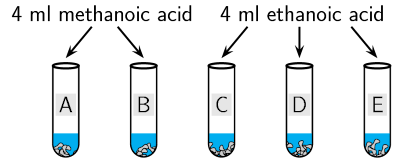4. Add $$\text{5}$$ $$\text{ml}$$ methanol to test tubes A and C.

5. Add $$\text{5}$$ $$\text{ml}$$ ethanol to test tubes B and D.

6. Add $$\text{5}$$ $$\text{ml}$$ pentanol to test tube E.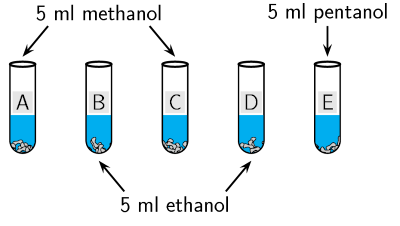7. Slowly add $$\text{2}$$ $$\text{ml}$$ $$\text{H}_{2}\text{SO}_{4}$$ to each test tube.

8. Soak the paper towel in cold water and attach it to the sides near the top of each test tube with a rubber band (do not close the test tube, just wrap the paper around the sides near the top).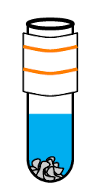9. Heat the water bath to $$\text{60}$$ $$\text{℃}$$ (using the hot-plate or bunsen burner) and place the test tubes in it for $$\text{10}$$-$$\text{15}$$ $$\text{min}$$.

10. After $$\text{10}$$-$$\text{15}$$ $$\text{min}$$ cool each test tube in cold water. Label the bowls of cold water A - E and pour the contents of test tube A into bowl A, etc.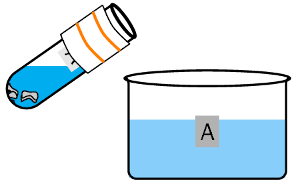11. Observe the surface of the water and note the smell in each bowl.

### Questions

1. What is the purpose of the $$\text{H}_{2}\text{SO}_{4}$$?

2. What is the purpose of the wet paper towel?

3. What did you observe on the top of the water in each bowl at the end?

4. Fill in the details in a table like the one below:

 Carboxylic acid Alcohol Product Smell methanoic acid methanol methanoic acid ethanol ethanoic acid methanol ethanoic acid ethanol ethanoic acid pentanol

### Discussion and Conclusion

An ester is the product of an acid-catalysed condensation between an alcohol and a carboxylic acid. Esters have identifiable aromas (like the fragrant smell and odour of fruit) and are used in perfumes. If you didn't smell anything it is likely that your water bath was set at too high a temperature and the ester degraded.

Esters are less water soluble than the carboxylic acid they were formed from and appear as an oily substance on water. Before being cooled however some of the ester would have been a vapour, so the wet paper towel would help to prevent a loss of the product.

Refer to Table 4.17 and see if the smells match those listed there. Remember to waft the smell towards you using your hand, do not sniff the fumes directly!

## Esters

Textbook Exercise 4.25

Give the IUPAC name for the product in the esterification of ethanoic acid with:

methanol

methyl ethanoate

octanol

octyl ethanoate

hexanol

hexyl ethanoate

propanol

propyl ethanoate

What is another name for the type of reaction in the question above?

acid-catalysed condensation

Give the IUPAC name for the product in the reaction of butanol with:

ethanoic acid

butyl ethanoate

pentanoic acid

butyl pentanoate

heptanoic acid

butyl heptanoate

methanoic acid

butyl methanoate

Fill in the missing reactant or part of the product name in the reactions below:

octanol + $$\_\_\_\_\_\_ \to \_\_\_\_\_\_$$ hexanoate

octanol + hexanoic acid $$\to$$ octyl hexanoate

$$\_\_\_\_\_\_$$ + propanoic acid $$\to$$ hexyl $$\_\_\_\_\_\_$$

hexanol + propanoic acid $$\to$$ hexyl propanoate

$$\_\_\_\_\_\_$$ + butanol $$\to \_\_\_\_\_\_$$ pentanoate

pentanoic acid + butanol $$\to$$ butyl pentanoate